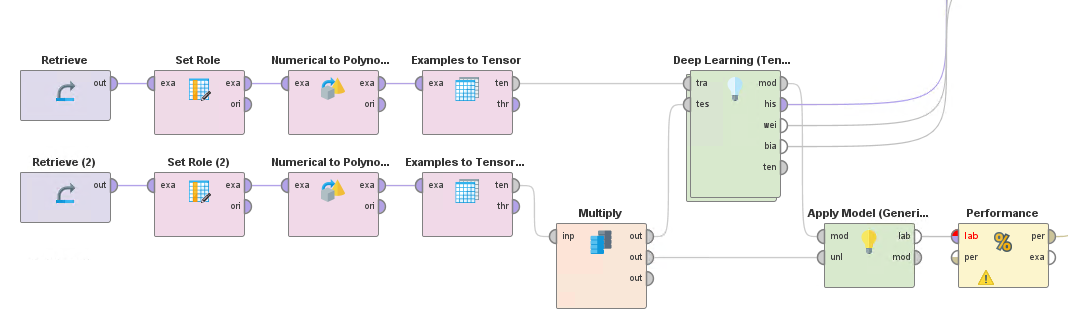# How to do Tensorflow image recognition?

Member Posts: 1Newbie
edited March 2021 in Help
I want to load "fashion_mnist" dataset from "tensorflow_datasets" and process it with "Deep Learning" extension.
The original "fashion_mnist" data is (60000,(28,28,1),(1)) so, I converted it to the BATCH/ID Tensor by "Execute Python":

```import pandas as pd
import numpy as np
import tensorflow as tf
import tensorflow_datasets as tfds
tfds.disable_progress_bar()
image_size = 28

def normalize_img(row):
img = row.image.reshape((image_size,image_size))/255.0
img = np.append(img, [range(image_size)], axis=0)
img = np.append(img, [np.ones(image_size)*row.name], axis=0)
img = np.append(img, [np.ones(image_size)*row.label], axis=0)
row.image = img.T
return row

def preprocess_df(df):
df.image = df.apply(normalize_img, axis=1)
df = pd.DataFrame(np.dstack(df.image.to_numpy()).transpose(2,0,1).reshape(df.shape*image_size,image_size+3))
df.rename(columns={28: 'id', 29:'batch', 30:'label'}, inplace=True)
return df

def rm_main():